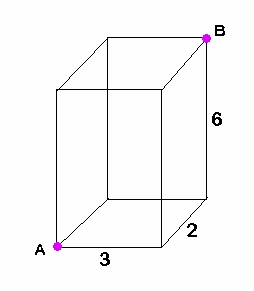# ACT, Geometry – diagonal of a rectangular prism

Q: What is the (straight-line) distance between points A and B on the figure below?Explanation:  We are finding the length of the green segment below:This requires the three-dimensional version of the distance formula:

There is another way to do this, using a progression of two right triangles, with the green segment being the hypotenuse of the second triangle, but I find the above formula much simpler.  It is just an extension of the ‘usual’ (2-dimensional) distance formula we are so familiar with from Algebra and Geometry.

BUT if you want an even easier way, and I know you do, use my TI-84 Plus program ‘DIST3D’.Lorem ipsum dolor sit amet, consectetur adipisicing elit, sed do eiusmod tempor incididunt ut labore et dolore magna aliqua. Ut enim ad minim veniam, quis nostrud exercitation ullamco laboris nisi ut aliquip ex ea commodo consequat.

# SAT, Geometry – triangles

Q: The lengths of two sides of a triangle are 4 and 6. If the third side is an integer, what is one possible value for the perimeter?

Answer:  The perimeter can be any of the following values: 13, 14, 15, 16, 17, 18, or 19.

Explanation: The length of the third side of any triangle must be longer than the difference of the other two sides, but shorter than the sum. (This is called the Triangle Inequality Theorem.) Here, the third side is longer than 6-4=2 and shorter than 6+4=10. It can be any integer between, but not including, 2 and 10. Recall that integers include only whole numbers and their negatives. The third side must then be either 3, 4, 5, 6, 7, 8, or 9. To get the possible perimeters, add the other two side lengths (4 and 6) to each of the possibilities for the third side. The result is that the perimeter can be 13, 14, 15, 16, 17, 18, or 19.